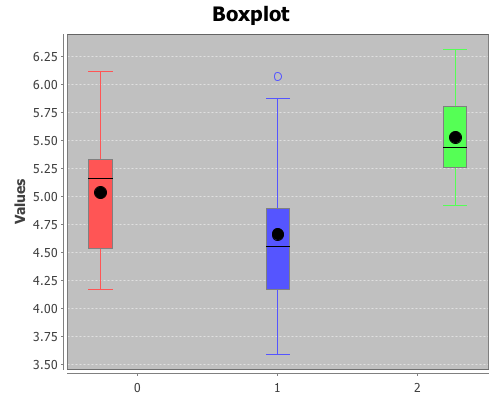# Student’s t-test

This example will demonstrate the t-test function for comparing the means of two samples. You will need the incanter.core, incanter.stats, incanter.charts, and incanter.datasets libraries.

``(use '(incanter core stats charts datasets))``

For more information on using these packages, see the matrices, datasets, and sample plots pages on the Incanter wiki.

Now load the plant-growth sample data set.

``(def plant-growth (to-matrix (get-dataset :plant-growth)))``

Break the first column of the data into three groups based on the treatment group variable (second column) using the group-by function,

``(def groups (group-by plant-growth 1 :cols 0))``

and print the means of the groups

``(map mean groups) ;; returns (5.032 4.661 5.526)``

View box-plots of the three groups.

``````(doto (box-plot (first groups))
view)``````

This plot can also be achieved using the :group-by option of the box-plot function.

``````(view (box-plot (sel plant-growth :cols 0)
:group-by (sel plant-growth :cols 1)))``````Create a vector of t-test results comparing the groups,

``````(def t-tests [(t-test (second groups) :y (first groups))
(t-test (last groups) :y (first groups))
(t-test (second groups) :y (last groups))])``````

Print the p-values of the three groups,

``(map :p-value t-tests) ;; returns (0.250 0.048 0.009)``

Based on these results the third group (treatment 2) is statistically significantly different from both the first group (control) and the second group (treatment 1). However, treatment 1 is not statistically significantly different than the control group.

The complete code for this example can be found here.

### 2 responses to “Student’s t-test”

1.Eurekin

Got following error while executing the code:
user=> (def groups (group-by plant-growth 1 :cols 1))
java.lang.IllegalArgumentException: Wrong number of args (4) passed to: core\$group-by (NO_SOURCE_FILE:4)

I’m using Incanter.exe from one of your latest posts.

•liebke

the method group-by was renamed to group-on after another method called group-by was added to clojure.core. Here’s a link to the documentation for group-on:

http://liebke.github.com/incanter/core-api.html#incanter.core/group-on